International
Tables for
Crystallography
Volume A1
Symmetry relations between space groups
Edited by Hans Wondratschek and Ulrich Müller

International Tables for Crystallography (2006). Vol. A1, ch. 1.4, p. 27   | 1 | 2 |

## Section 1.4.2. Basic capabilities of the Cryst package

Franz Gählera*

aInstitut für Theoretische und Angewandte Physik, Universität Stuttgart, D-70550 Stuttgart, Germany
Correspondence e-mail: gaehler@itap.physik.uni-stuttgart.de

### 1.4.2. Basic capabilities of the Cryst package

| top | pdf |

Before we describe in more detail the checks that have been performed, we briefly summarize the capabilities of the Cryst package and how space groups are handled by Cryst.

In Cryst, a space group is represented as a fixed group of augmented matrices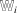, cf. Section 1.2.2.4. The group multiplication therefore coincides with matrix multiplication. The space group is defined by an arbitrary (finite) set of generating elements. The space group consists of the set of all augmented matrices that can be obtained as products of the generating matrices. It is not important which generating elements are chosen. Different sets of generators can define the same space group.

The representation of a space group as a group of augmented matrices implicitly requires the choice of an origin and a vector basis of Euclidean space. The Cryst package does not require any particular choice of origin or vector basis. In particular, it is not necessary to work with a lattice basis; in fact, any basis can be used. This has the advantage that one can continue to use the basis chosen for the parent group when passing to a subgroup.

Space groups are conjugated by some affine coordinate transformation if they differ only in their settings, i.e. by the choice of their origins and/or vector bases. Such an affine coordinate transformation is also represented by an augmented matrix. Given a coordinate transformation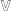and a space group, the transformed space group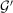is simply generated by the transformed generators of.

If a space groupis given by a set of generators, Cryst first needs to compute the point group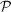of, and the translation subgroup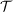, represented by a (canonical) basis of the translation lattice (the basis of the translation lattice chosen by Cryst is canonical in the sense that the same basis is always chosen for a given). The point groupis simply generated by the linear parts of the generators of. The mapping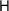, which sends each element ofto its linear part, is in fact a group homomorphism:. The point groupis finite and each point-group element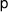can easily be expressed as a product of generators of, which are images of generators ofunder. This provides a way to compute, for any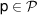, some representative pre-image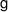ofunder, i.e. some element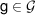which is mapped ontoby. Having expressed the elementas a product of generators, one simply replaces the factors in this product by their representative pre-images under, which are known by construction, to obtain the representative pre-imageof.

To compute a generating set of the translation subgroup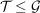, one first computes a set of defining relations of the point groupfor the given generators. For a finite group this is a standard task in GAP. These defining relations are a set of inequivalent ways to express the identity element ofby the generators of. Replacing the factors in such a defining relation by their representative pre-images inyields a pre-image of the identity of, i.e. an element of. The translations so obtained generate, together with the pure translation generators of, the entire translation subgroup.

We now have all the necessary information to test whether a given augmented matrix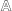is an element of a space group. One first tests whether the linear part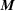ofis an element of the point groupof. If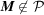,is not an element of. Otherwise, some pre-imageofinis computed.is then an element ofif and only ifanddiffer in their translation part by an element of the translation group. With this membership test, we can determine whether two space groups are equal, or whether one is a subgroup of the other: a space group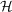is a subgroup of a space groupif all the generators ofare elements of. Two space groups are equal if either of them is a subgroup of index 1 of the other.